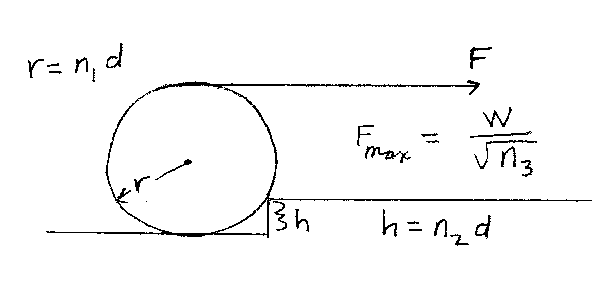Problem A15: In the figure below, a circular solid disk is leaning up against a step. The radius of the disk is r=n1d. The height of the step is h=n2d. A force of magnitude F is applied to a rope that is attached to the top of the disk. The rope pulls horizontally. The disk has a weight W. What is the maximum force that can be applied such that the disk is in static equilibrium as shown in the figure. If the maximum force is F=W/ √ n3 , what is n3?n1 = n2 = Input n3:

If you are currently in my class, you can record your grade by entering your name and student ID number (without the leading zeros) below and clicking on "record grade".
 First Name = Last Name = ID = Problem: